CBSE Class 12 Sample Paper for 2023 Boards

Class 12
Solutions of Sample Papers and Past Year Papers - for Class 12 Boards

## r = 20i ̂-10j ̂+4k ̂+μ(10i ̂-20j ̂+10k ̂ )?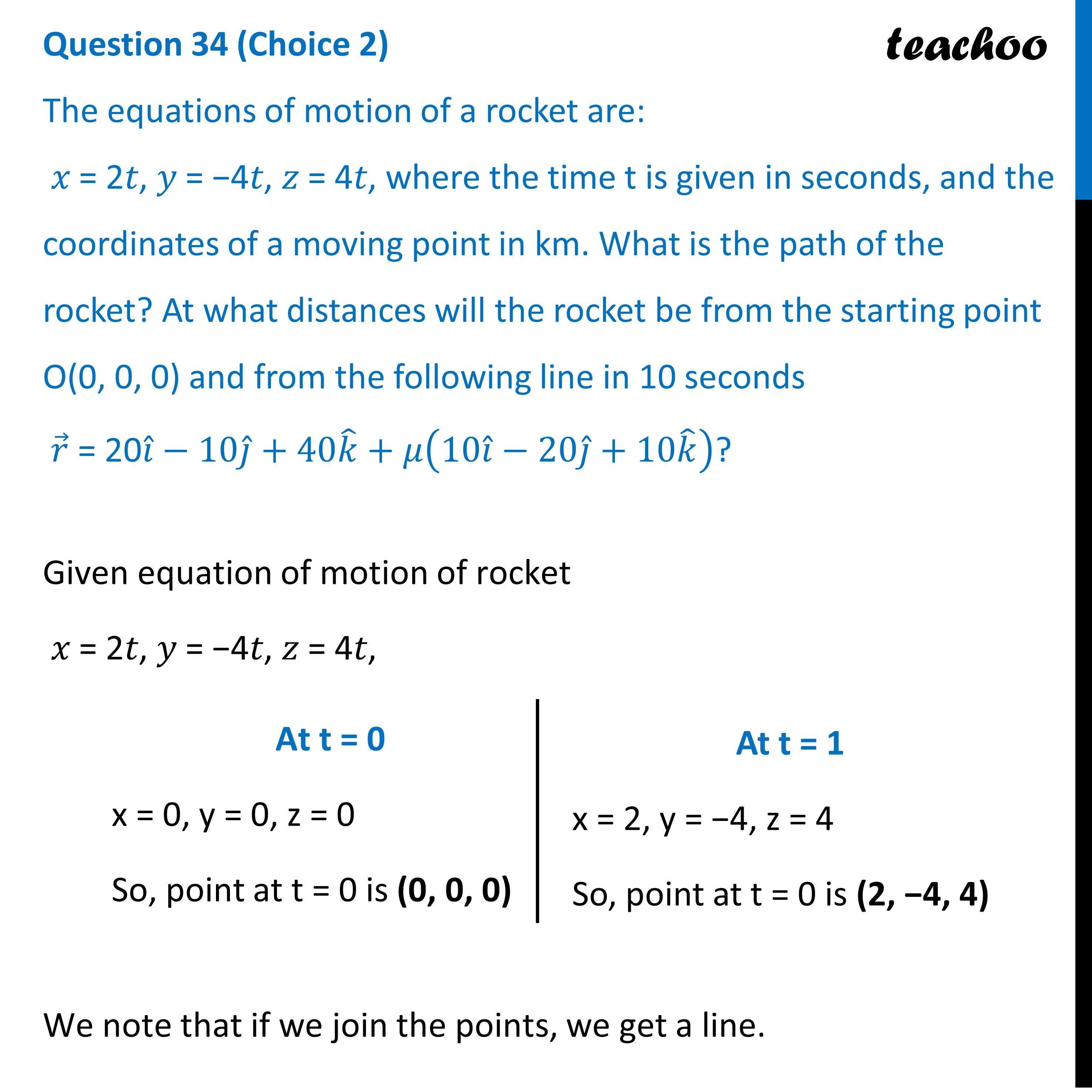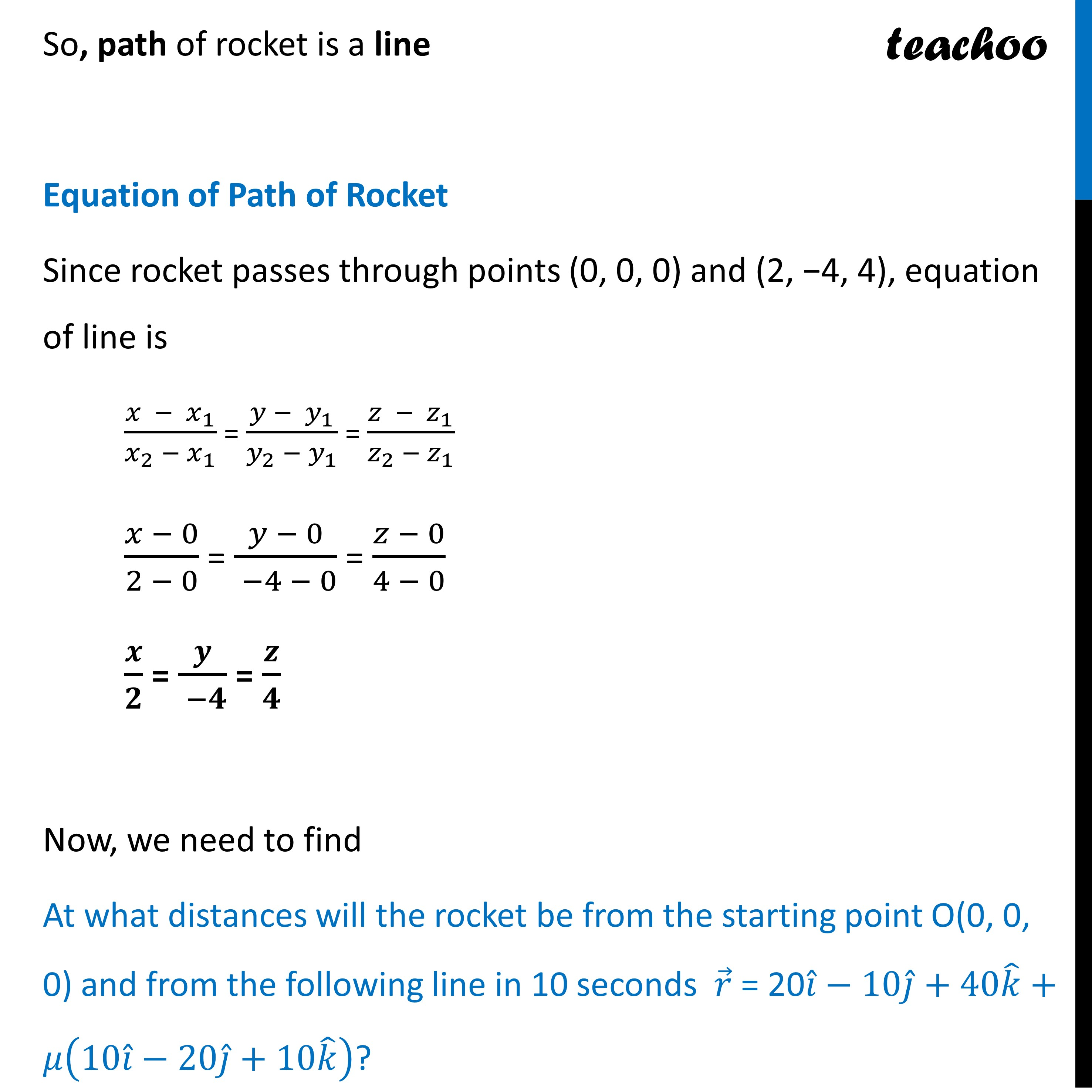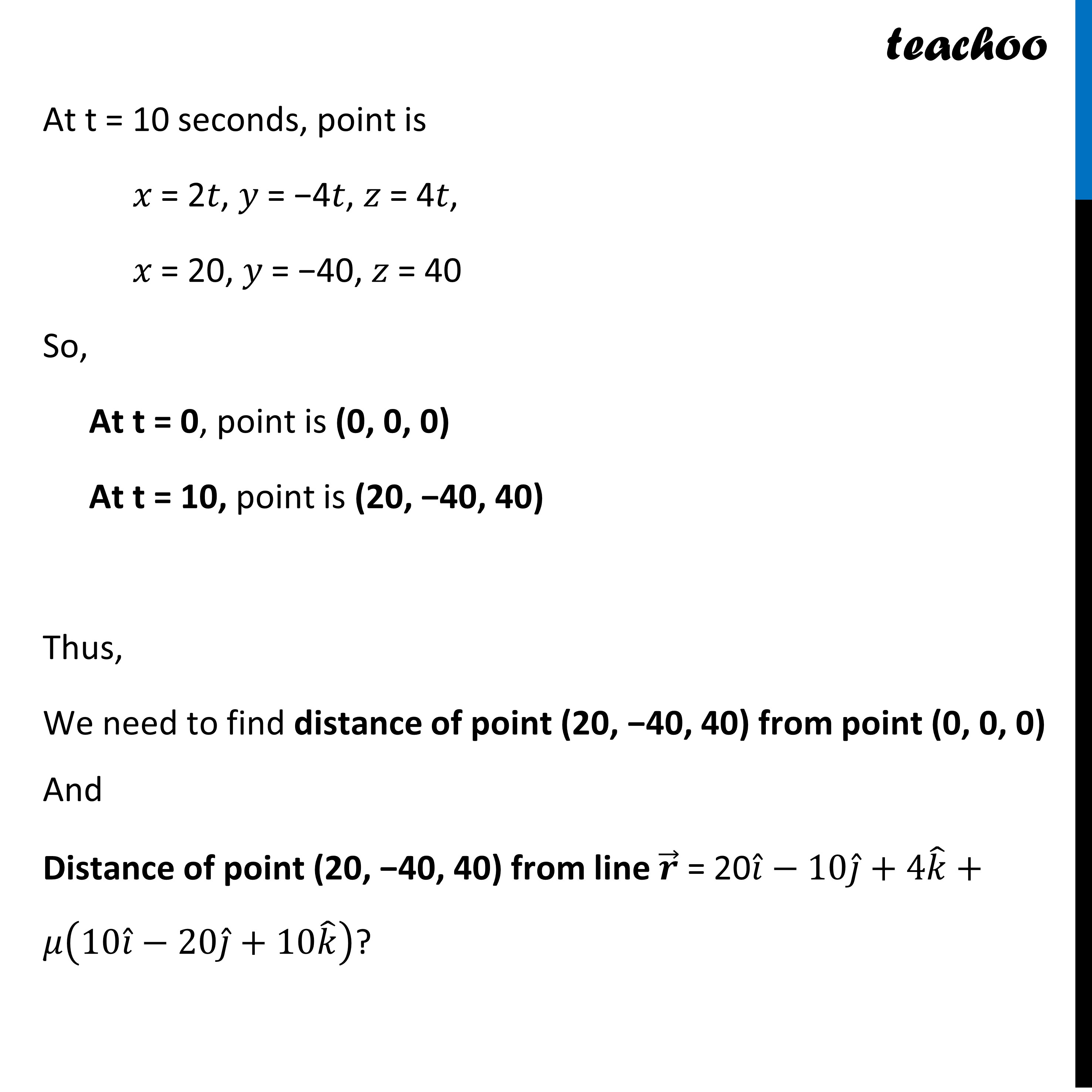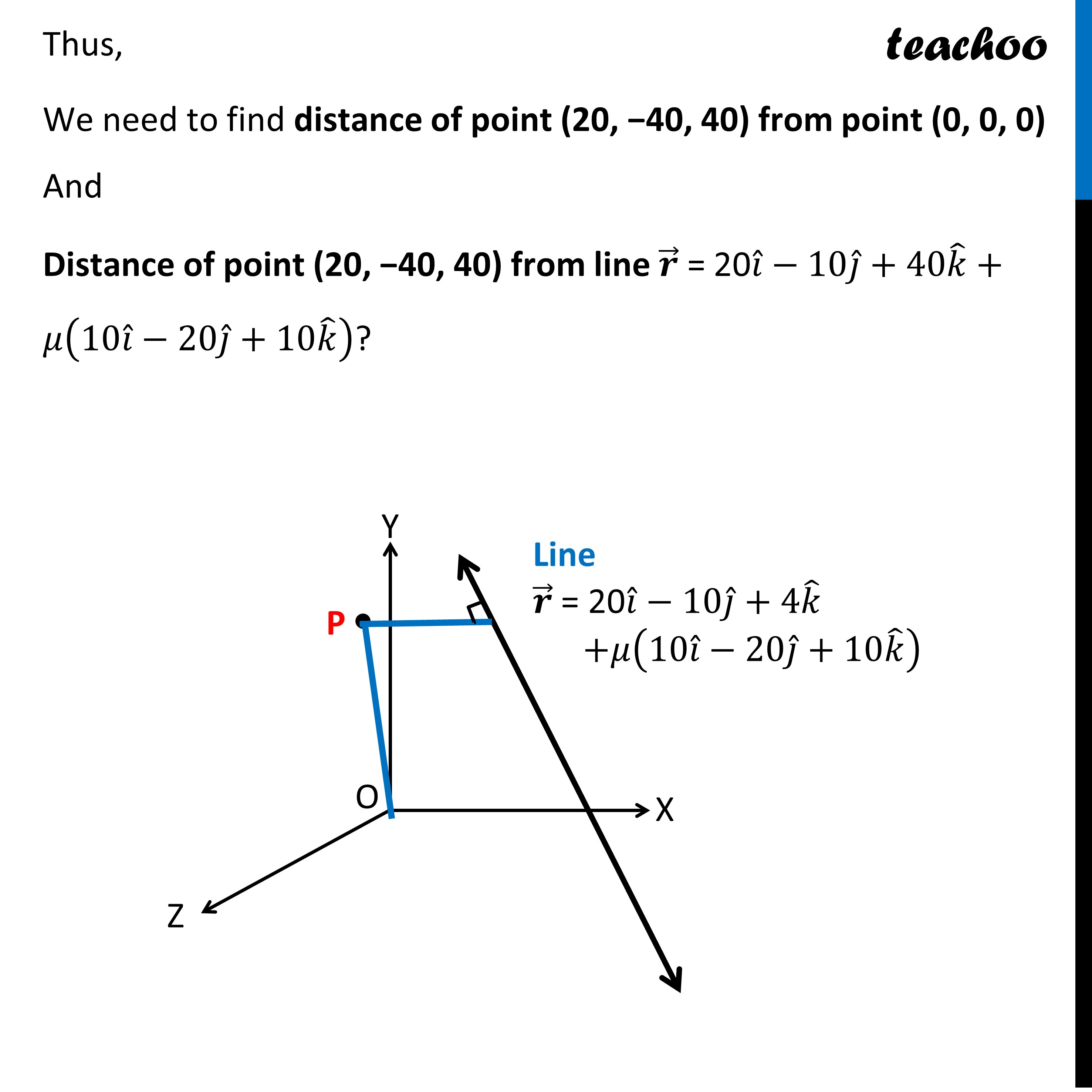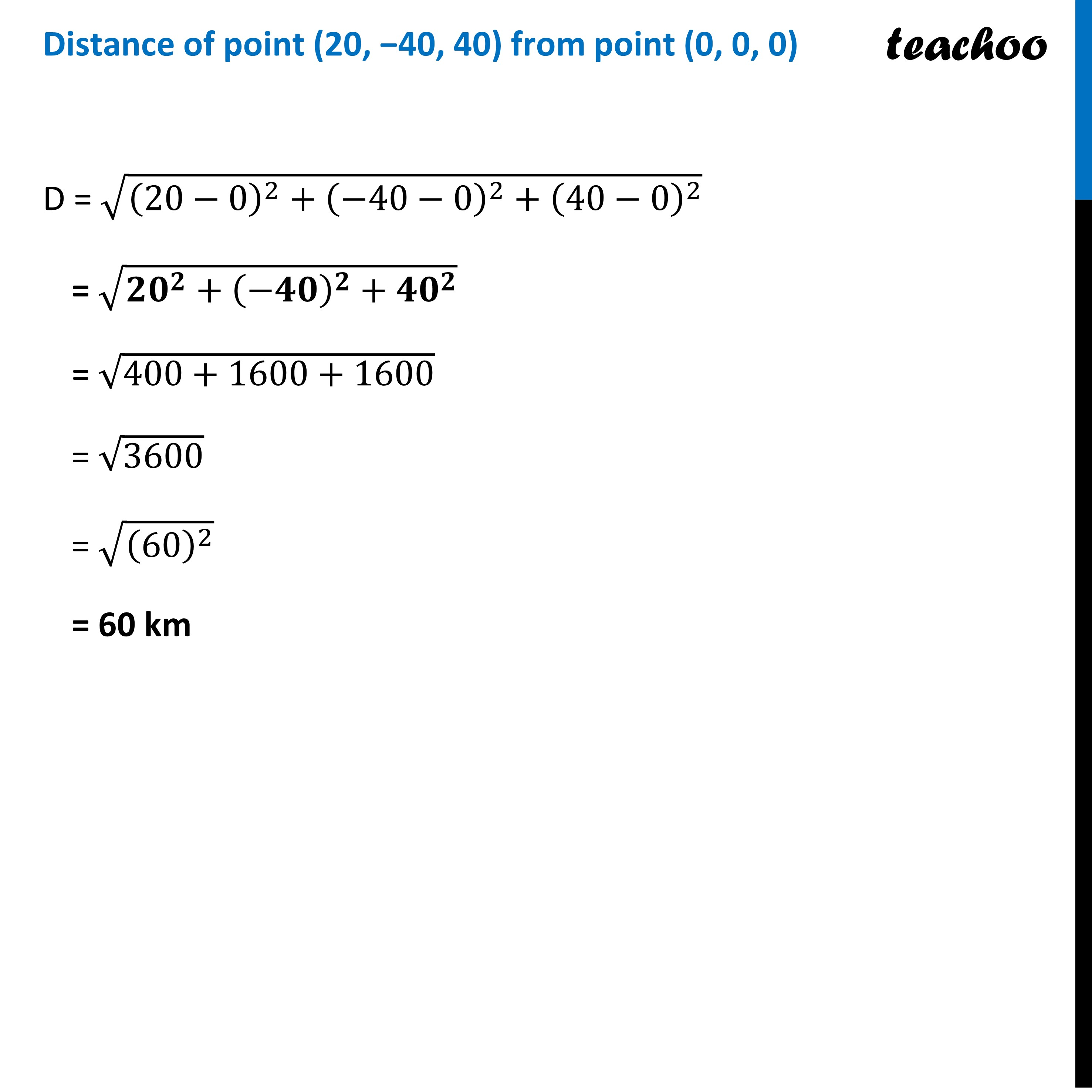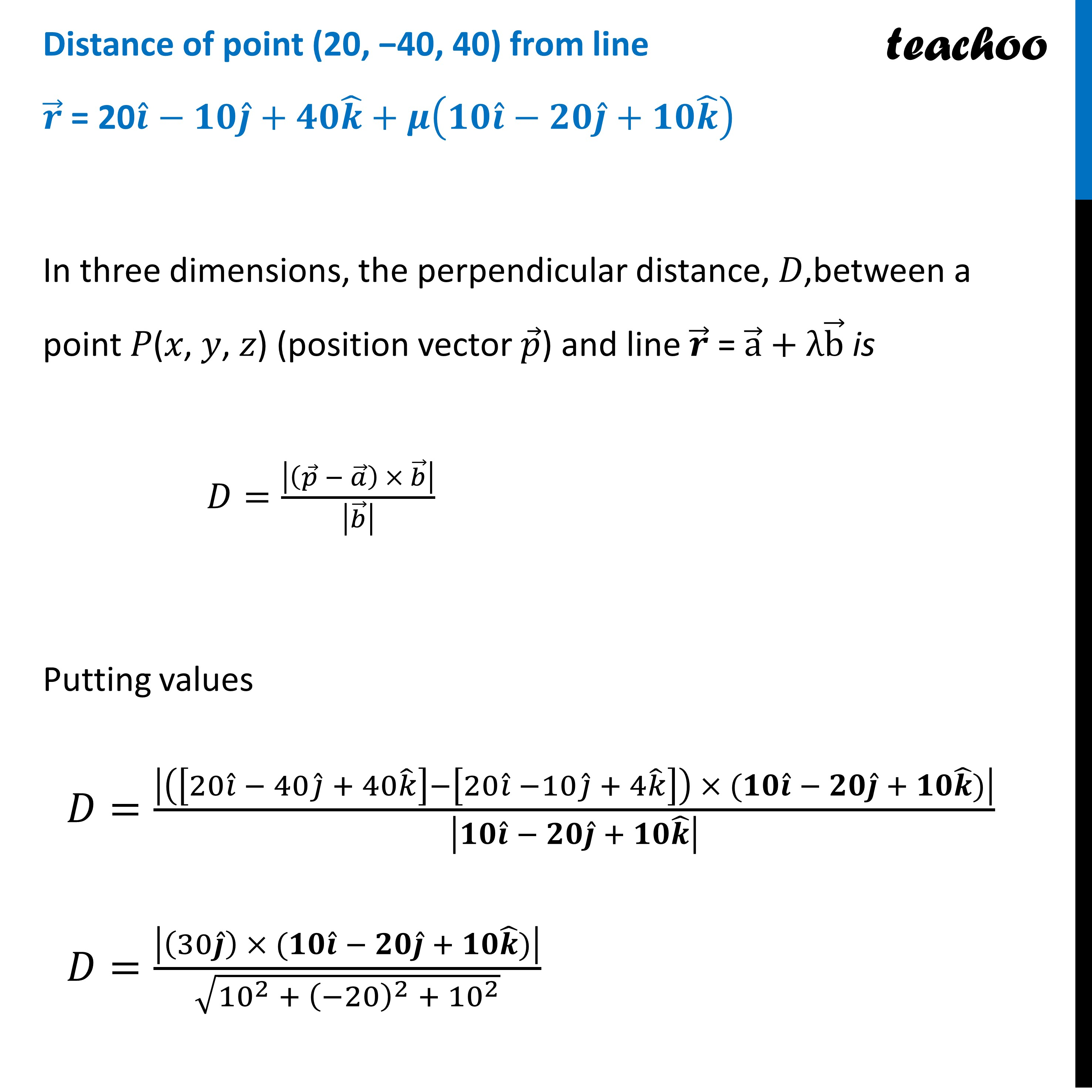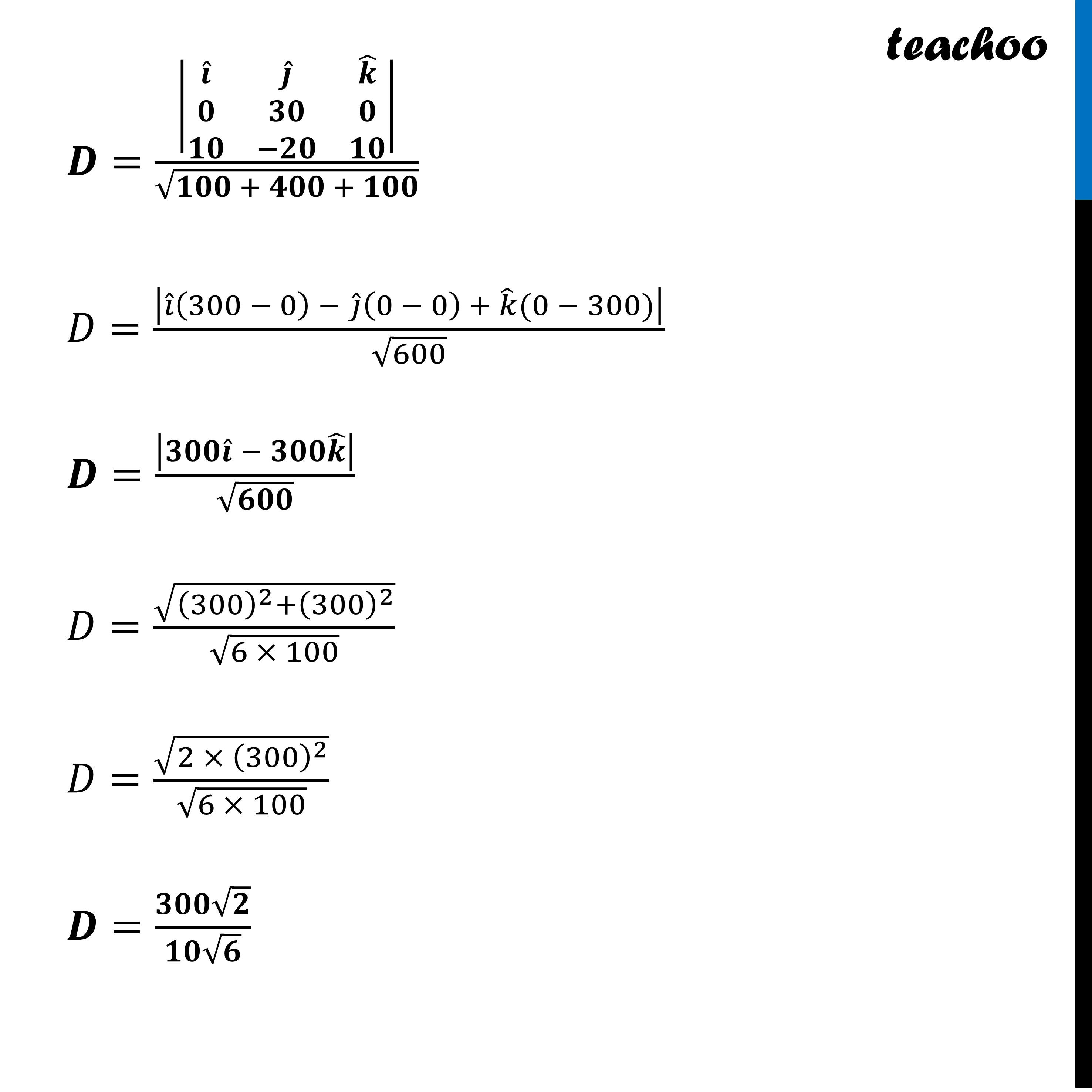Learn in your speed, with individual attention - Teachoo Maths 1-on-1 Class

### Transcript

Question 34 (Choice 2) The equations of motion of a rocket are: 𝑥 = 2𝑡, 𝑦 = −4𝑡, 𝑧 = 4𝑡, where the time t is given in seconds, and the coordinates of a moving point in km. What is the path of the rocket? At what distances will the rocket be from the starting point O(0, 0, 0) and from the following line in 10 seconds 𝑟 ⃗ = 20𝑖 ̂−10𝑗 ̂+40𝑘 ̂+𝜇(10𝑖 ̂−20𝑗 ̂+10𝑘 ̂ )?Given equation of motion of rocket 𝑥 = 2𝑡, 𝑦 = −4𝑡, 𝑧 = 4𝑡, We note that if we join the points, we get a line. At t = 0 x = 0, y = 0, z = 0 So, point at t = 0 is (0, 0, 0) At t = 1 x = 2, y = −4, z = 4 So, point at t = 0 is (2, −4, 4) So, path of rocket is a line Equation of Path of Rocket Since rocket passes through points (0, 0, 0) and (2, −4, 4), equation of line is (𝑥 − 𝑥_1)/(𝑥_2 − 𝑥_1 ) = (𝑦 − 𝑦_1)/(𝑦_2 − 𝑦_1 ) = (𝑧 − 𝑧_1)/(𝑧_2 − 𝑧_1 ) (𝑥 − 0)/(2 − 0) = (𝑦 − 0)/( −4 − 0) = (𝑧 − 0)/(4 − 0) 𝒙/𝟐 = 𝒚/( −𝟒) = 𝒛/𝟒 Now, we need to find At what distances will the rocket be from the starting point O(0, 0, 0) and from the following line in 10 seconds 𝑟 ⃗ = 20𝑖 ̂−10𝑗 ̂+40𝑘 ̂+𝜇(10𝑖 ̂−20𝑗 ̂+10𝑘 ̂ )? At t = 10 seconds, point is 𝑥 = 2𝑡, 𝑦 = −4𝑡, 𝑧 = 4𝑡, 𝑥 = 20, 𝑦 = −40, 𝑧 = 40 So, At t = 0, point is (0, 0, 0) At t = 10, point is (20, −40, 40) Thus, We need to find distance of point (20, −40, 40) from point (0, 0, 0) And Distance of point (20, −40, 40) from line 𝒓 ⃗ = 20𝑖 ̂−10𝑗 ̂+4𝑘 ̂+𝜇(10𝑖 ̂−20𝑗 ̂+10𝑘 ̂ )? Thus, We need to find distance of point (20, −40, 40) from point (0, 0, 0) And Distance of point (20, −40, 40) from line 𝒓 ⃗ = 20𝑖 ̂−10𝑗 ̂+40𝑘 ̂+𝜇(10𝑖 ̂−20𝑗 ̂+10𝑘 ̂ )? Line 𝒓 ⃗ = 20𝑖 ̂−10𝑗 ̂+4𝑘 ̂ +𝜇(10𝑖 ̂−20𝑗 ̂+10𝑘 ̂ ) Distance of point (20, −40, 40) from point (0, 0, 0) D = √((20−0)^2+(−40−0)^2+(40−0)^2 ) = √(〖𝟐𝟎〗^𝟐+(−𝟒𝟎)^𝟐+〖𝟒𝟎〗^𝟐 ) = √(400+1600+1600) = √3600 = √((60)^2 ) = 60 kmDistance of point (20, −40, 40) from line 𝒓 ⃗ = 20𝒊 ̂−𝟏𝟎𝒋 ̂+𝟒𝟎𝒌 ̂+𝝁(𝟏𝟎𝒊 ̂−𝟐𝟎𝒋 ̂+𝟏𝟎𝒌 ̂ ) In three dimensions, the perpendicular distance, 𝐷,between a point 𝑃(𝑥, 𝑦, 𝑧) (position vector 𝑝 ⃗) and line 𝒓 ⃗ = a ⃗ + λb ⃗ is 𝐷=|(𝑝 ⃗ − 𝑎 ⃗ ) × 𝑏 ⃗ |/|𝑏 ⃗ | Putting values 𝐷=|([20𝑖 ̂ − 40𝑗 ̂ + 40𝑘 ̂ ]−[20𝑖 ̂ −10𝑗 ̂ + 4𝑘 ̂ ]) × (𝟏𝟎𝒊 ̂ − 𝟐𝟎𝒋 ̂ + 𝟏𝟎𝒌 ̂)|/|𝟏𝟎𝒊 ̂ − 𝟐𝟎𝒋 ̂ + 𝟏𝟎𝒌 ̂ | 𝐷=|(30𝒋 ̂ ) × (𝟏𝟎𝒊 ̂ − 𝟐𝟎𝒋 ̂ + 𝟏𝟎𝒌 ̂)|/√(〖10〗^2 + (−20)^2 + 〖10〗^2 ) 𝑫=|■8(𝒊 ̂&𝒋 ̂&𝒌 ̂@𝟎&𝟑𝟎&𝟎@𝟏𝟎&−𝟐𝟎&𝟏𝟎)|/√(𝟏𝟎𝟎 + 𝟒𝟎𝟎 + 𝟏𝟎𝟎) 𝐷=|𝑖 ̂(300 − 0) − 𝑗 ̂(0 − 0) + 𝑘 ̂(0 − 300)|/√600 𝑫=|𝟑𝟎𝟎𝒊 ̂ − 𝟑𝟎𝟎𝒌 ̂ |/√𝟔𝟎𝟎 𝐷=√((300)^2+(300)^2 )/√(6 × 100) 𝐷=√(2 × (300)^2 )/√(6 × 100) 𝑫=(𝟑𝟎𝟎√𝟐)/(𝟏𝟎√𝟔) 𝐷=30/√3 𝐷=30/√3 ×√3/√3 𝐷=(30√3)/3 D = 10 √𝟑 km